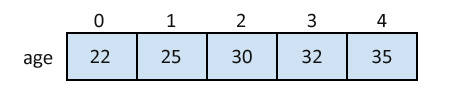## C Programming Tutorial Index

The array is a data structure in C programming that can store a fixed-size sequential collection of elements of the same data type. In this tutorial, you will learn to use arrays in C programming.

For example, if you want to store ten numbers, it is easier to define an array of 10 lengths instead of ten variables.

In the C programming language, an array can be One-Dimensional, Two-Dimensional, and Multidimensional.

## Define an Array in C

Syntax:

``type arrayName [ size ];``

This is called a one-dimensional array. An array type can be any valid C data type, and the array size must be an integer constant greater than zero.

Example:

``double amount;``

## Initialize an Array in C

Arrays can be initialized at declaration time:

Example:

``int age = {22,25,30,32,35};``

Initializing each element separately in a loop:

``````int myArray;
int n = 0;

// Initializing elements of the array separately.
for(n=0;n<sizeof(myArray)/sizeof(myArray);n++)
{
myArray[n] = n;
}``````

### A Pictorial Representation of the Array:## Accessing Array Elements in C

Example:

``````int myArray;
int n = 0;

// Initializing elements of array seperately.
for(n=0;n<sizeof(myArray)/sizeof(myArray);n++)
{
myArray[n] = n;
}

int a = myArray; // Assigning 3rd element of array value to integer 'a'.``````

### C Arrays - Video Tutorial

Please watch this video tutorial to understand "C Arrays" in depth.